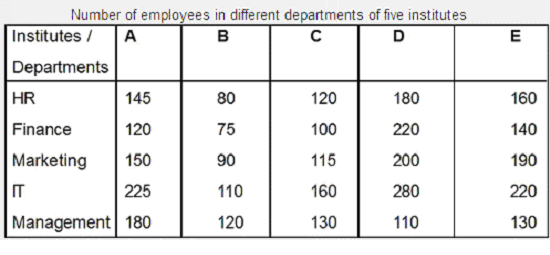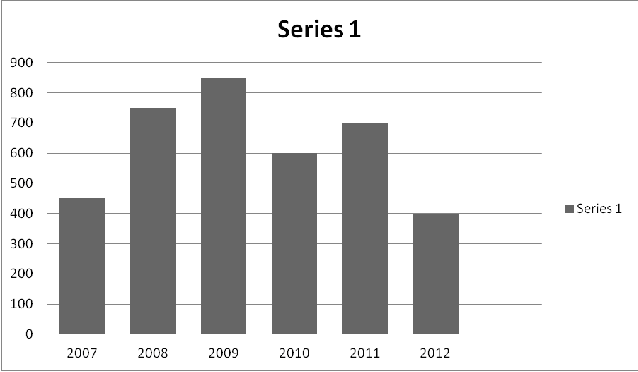# IBPS Quantitative Aptitude model test paper - 5

Home > Aptitude test > Quantitative model test - 5

## IBPS Quantitative Aptitude model test paper - 5

Directions (Q.1-5) What value should come in place of the question mark (?) in the following question?

Q1. √196 + √961 = ?

A. 15
B. 35
C. 55
D. 45

Q2. 1948 ÷ 52 + 10240 ÷ 20 + 512 - 248 -520.3615 = ?

A. 20.93
B. 293
C. 200.93
D. 201

Q3. 4.789 x 3.14 x 5.25 - ? = 0.145 x 0.111 x 121

A. 76.999
B. 812.55
C. 718.98
D. 178

Q4. 10.5% of 1000 - 8.5% of 100 + 3.5 = ?

A. 10
B. 110
C. 100
D. 120

Q5. 1/5 of 3/3 of 1/4 of 30000 = ?

A. 1500
B. 150
C. 300
D. 30

Q6. 7 person can do a piece of work in 21 days. How many persons are required to do a piece of work in 3 days?

A. 48
B. 49
C. 41
D. 42

Q7. Sam got 33 marks in Civil, 35 marks in Electrical, 38 marks in Maths, 28 marks in Electronics ad 26 in Philosophy. A student can get maximum 40 marks in one subject. What is the

percentage of Sam in the exam?

A. 90
B.95
C. 97
D. 80

Q8. What will come in place of question mark (?) in the following number series?

720 120 24 6 2 ?

A. 2
B. 1
C. 0
D. None of these

Q9. What will come in place of question mark (?) in the following number series?

26.5 29 27 ? 27.5 28

A. 28
B. 28.5
C. 27.2
D. 26.5

Q10. The following questions are accompanied three statement a, b & c. You have to determine which statement(s) is /are necessary sufficient to answer the question?

Ram, Shyam and Prakash together will finish the work in how many days?

a. Ram does half as much work as Shyam and Shyam does half as much work as Ram and Shyam does together in one day.
b. Shyam does the whole work in 40 days.
c. Ram and Shyam do the whole work in 20 days.

A. Only a or b or c
B. Either a and b or a and c
C. Only b and c
D. Only a

ANSWER: B. Either a and b or a and c

Q11. What approximate value will come in the place of question mark (?) in the following questions .

2228 = ?

A. 43
B. 49
C. 84
D. 86
E. None of these

Directions (Q. 12-16) Study the following table carefully and answer the questions given below:Q12. The number of employees in IT department in institute E is approximately what percent more than that in Finance department?

A. 57
B. 42
C. 17
D. 66

Q13. The number of employees in IT department of institute B is approximately what percent of the total number of employees in the same institute?

A. 33
B. 29
C. 23
D. 14

Q14. What is the respective ratio between the number f employees in marketing and management departments in all institutes?

A. 11:7
B. 149:134
C. 117:119
D. 3 : 29

Q15. What is the total number of employees in all the departments in institute D?

A. 999
B. 936
C. 978
D. None of these

Q16. What is the average number of employees in Finance department in all the institutes?

A. 143
B.175
C. 134
D. 131

Directions (Q. 17-18). In the following questions two equations numbered I and II are given. You have to solve both the equations and give answer

1) If a> b
2) If a>= b
3) If a < b
4) If a=5) If a= b or the relationship cannot be established

Q17. I. 5a2 = 18a – 9.
II. b3 = 1331

A. Only 1 follows
B. Only 3 follows
C. Only 5 follows
D. Only 4 follows.

Q18. I. (a+155) 1/2 – (36) 1/2 = (49) 1/2
II. b2 = 121

A. Only 1 follows
B. Only 3 follows
C. Only 5 follows
D. Only 4 follows.

Q19. A bag contains six eggs and eight potatoes. One article is drawn at random. What is the probability that the article drawn is potato?

A. 1/8
B. 6/8
C. 4/7
D. None of these.

Q20. Hari invested an amount of Rs. 16000 in a fixed deposit scheme for 3 years at Compound interest rate 5% per annum. How much amount will Hari het on maturity of the fixed deposit ?

A. 19500
B. 8820
C. 18522
D. None of these

Directions (Q21. 25) Study the following bar-graph and answer the questions given below:
Production of Milk by a company (in 1000 litres) over the years.Q21. In how many of the given years was the production of Milk more than the average production of the given years?

A. 1
B. 2
C. 3
D. 4

Q22. The average production of 2007 and 2008 was exactly equal to the average production of which of the following pairs of years?

A. 2007 & 2011
B. 2008 & 2012
C. 2009 & 2010
D. None of these

Q23. What was the percentage decline in the production of milk from 2011 to 2012?

A. 42.85%
B. 36.36%
C. 57.14%
D. 58.15%

Q24. In which year was the percentage increase in production as compared to the previous year the maximum?

A. 2009
B. 2011
C. 2007
D. 2008

Q25. What was the percentage increase in production of Milk in 2011 compared to that of 2007?

A. 125%
B. 55.55%
C. 75.25%
D. None of these.